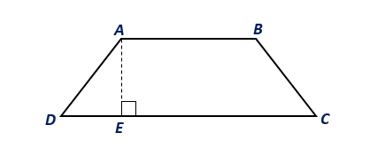Chapter 8.CT, Problem 8CT### Elementary Geometry for College St...

6th Edition
Daniel C. Alexander + 1 other
ISBN: 9781285195698

#### Solutions

Chapter
Section### Elementary Geometry for College St...

6th Edition
Daniel C. Alexander + 1 other
ISBN: 9781285195698
Textbook Problem
1 views

# In trapezoid ABCD, A B = 7 ft and D C = 13 ft. If the area of trapezoid ABCD is 60 ft2, find the length of altitude A E ¯ .                                   _To determine

To find:

The length of the altitude AE¯ of the trapezoid ABCD.

Explanation

Given:

In trapezoid ABCD, AB=7 ft and DC=13 ft., and the area of trapezoid ABCD is 60 ft2, find the length of altitude AE¯.

Theorem:

The area A of a trapezoid whose bases have lengths b1 and b2 and whose altitude has length h is given by A=12h(b1+b2).

From the given, in trapezoid ABCD, AB=7 ft., and DC=13 ft., and area of ABCD is 60 ft2.

Let b1=7 ft

### Still sussing out bartleby?

Check out a sample textbook solution.

See a sample solution

#### The Solution to Your Study Problems

Bartleby provides explanations to thousands of textbook problems written by our experts, many with advanced degrees!

Get Started

#### In Exercises 33-38, rewrite the expression using positive exponents only. 33. (xy)2

Applied Calculus for the Managerial, Life, and Social Sciences: A Brief Approach

#### For f(x) = ex x, f(x) = a) ex x b) ex 1 c) e1 x d) e1 1

Study Guide for Stewart's Single Variable Calculus: Early Transcendentals, 8th

#### Graph: f(x)=4x2+1.

College Algebra (MindTap Course List)